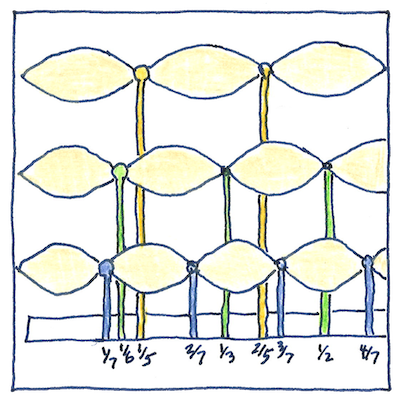# 1637

## The book of science

Tom Sharp

 Pythagoras, Vincenzo Galilei, Marin Mersenne acoustics

## Mersenne’s laws

• Pythagoras understood the first law of vibrating strings—
• pitch is inversely proportional to the length of the string.
• Pythagoras also knew that the series of harmonic overtones
• were related to the series of whole-number multiples.
• Moving right along, it turned out that musicians
• knew how to tune their lutes without Pythagoras.
• Galileo’s father, Vincenzo, a musician, certainly realized
• that pitch also depends on how tight and how thick the string is.
• He was the first to describe a natural phenomenon
• using a non-linear mathematical equation.
• Specifically, he added the second law of vibrating strings—
• pitch is proportional to the square root of the tension.
• Finally, Marin Mersenne added the third law—
• pitch is inversely proportional to the square root of the heaviness of the string.

## Acoustics

• Vitruvius wrote a treatise
• on the acoustics of theaters,
• recognizing that sound is a three-dimensional
• equivalent of waves on a water’s surface.
• Vincenzo Galilei, in addition
• to explaining the second law,
• noted that pitch depends on the properties
• of the space the string vibrates in.
• Galileo Galilei said, “Waves are produced by the vibrations
• of a sonorous body, which spread through the air,
• bringing to the tympanum of the ear a stimulus
• which the mind interprets as sound.”
• Isaac Newton’s inverse-square law
• applies to sound as well as gravity.
• In Principia Mathematica, Newton also
• derived the relationship for wave velocity in solids.

## Noise-to-signal ratio

• I can hear you
• in this restaurant
• but I don’t know what
• you’re saying.
• A great roar
• arises from little mouths
• talking louder
• to be heard over the roar.
• We vibrate
• our vocal chords
• which vibrate air
• in each other’s direction
• but our lips
• are a better
• indicator
• of our accord.

Marin Mersenne published L’Harmonie Universelle in 1637. The work of Pythagoras and Vincenzo Galilei, of course, was earlier. Pythagoras died around 495 BCE, and Galilei died in 1591. Some sources say that Galileo Galilei, independently of Mersenne and about the same time, discovered all three laws of vibrating strings.

By “a non-linear mathematical equation” we mean that the equation relates two things that are not merely directly or inversely proportional to each other, but that depend on the square or square root, cube or cube root.

See also in The book of science:

Readings on wikipedia: